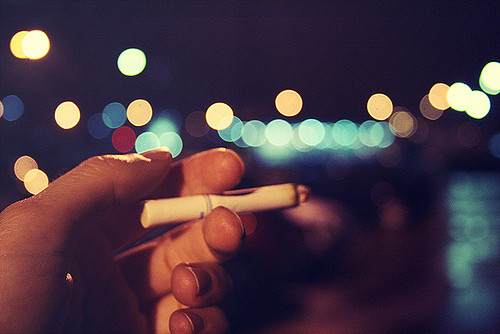### Creating an Interactive Visualization With BokehLast post, I created a model that would predict what effect changing the taxes on cigarettes would have on health care expenditures. That model was fun to build, but it is difficult to get a feel for what is going on. Even though it is a relatively simple model. Humans tend to be fairly visual creatures, so visualizing results is fairly important. In this post, what I want to do is to create an interactive visualization of the model. Specifically, I want to make something that a user could interact with and thus play with what the model is telling us about the world.

The tool that I chose to use was bokeh. Bokeh is not just a photography technique. It is a really neat little python package that let’s you visualize data. But more importantly, bokeh also let’s you create dynamic web based visualizations. The end result of this experiment in dynamic visualizations is a lightweight web app that is hosted on heroku for free. This post is the the second of three. In this post, I’m going to go over how to build the web app, and in the next I’ll go over how to deploy it on heroku.

### Building a Bokeh App

So we’ll be using three data sources for this app. The two we used in the last post, and the resulting trace from the last post that I pickled for later use. What we are going to do is create a plot of the tax rate for a selected state. The corresponding health care expenditures for that state, and a histogram of the distribution of simulated savings in health care expenditures that comes from the model that we built in the last post.

So how do we go about doing this. Assuming that you are working in the same environment as the last post, and that you still have all of the variables and datasets available to you, we’ll just jump into building the web app.

First import what we need into the python environment.

from bokeh.io import output_file, show
from bokeh.layouts import widgetbox,column,row
from bokeh.models.widgets import RadioGroup, Button, Dropdown, Select
from bokeh.models import Range1d
from bokeh.plotting import figure, curdoc
import pandas as pd
import pickle
import re
import numpy as np


With this stuff in the environment let’s make some plots. We’ll need three plots. We’ll also define some helper variables that we will make use of when we need to update the plot.

p1 = figure(x_range=[2005,2009], y_range=(0,1), title='Tax Rate Per Year')
p2 = figure(x_range=[2005,2009], y_range=(df['Data_Value'].min(),df['Data_Value'].max()),title='Expenditures on Cigarette Related Healthcare')
p3 = figure(title='Distribution of Savings (in Millions of \$) Per 1% Increase in Tax Rate per Year')
lines = []
lines2 = []
hists = []
i = 0
line_dict = {}
height_dict ={}
x_start={}
x_end = {}


Now that the basics are out of the way. We need to build a plot for each of the three graphs, and we need to make sure, that we can access them individually. So we’ll build the plots inside of a for-loop and keep track of which state we are working with using a dictionary. Note that for the histograms we are randomly pulling from the trace with replacement.

for state in df['LocationAbbr'].unique():
line_dict[state] = i
temp = df[df['LocationAbbr'] == state]
amt = temp.iloc[4,10]
hist, edges = np.histogram(np.random.choice((-trace)*amt,1000),density=True)
x_start[state] = edges
x_end[state] = edges[-1]
height_dict[state] = np.max(hist)
lines.append(p1.line(temp['Year'],temp['tax']))
lines2.append(p2.line(temp['Year'],temp['Data_Value']))
fill_color="#036564", line_color="#033649"))

i+=1


The next thing that we’ll do is kind of weird. We’re going to make all of the lines, and fills in the figures invisible.

for line in lines:
line.glyph.line_alpha=0
for line in lines2:
line.glyph.line_alpha=0
for hist in hists:
hist.glyph.fill_alpha=0
hist.glyph.line_alpha=0


The penultimate thing that we need to do is to define a callback function that will turn on the appropriate lines when we make a selection for which state we want to look at. This function turns off the old lines and turns on the new lines for each of the figures. That is all that it does.

def callback(attr, old, new):
p3.x_range.start=x_start[new]
p3.x_range.end=x_end[new]
p3.y_range.start=0
p3.y_range.end=height_dict[new]
lines[line_dict[old]].glyph.line_alpha=0
lines[line_dict[new]].glyph.line_alpha = 1
lines2[line_dict[old]].glyph.line_alpha=0
lines2[line_dict[new]].glyph.line_alpha = 1
hists[line_dict[old]].glyph.line_alpha=0
hists[line_dict[new]].glyph.line_alpha=1
hists[line_dict[old]].glyph.fill_alpha=0
hists[line_dict[new]].glyph.fill_alpha=1


With the callback function in place we just need to give ourselves a way to pick which state we want to look at. We’ll do this through a dropdown menu embedded in the app. Now the tricky thing here is to get all the available options in to the dropdown. We’ll do it by just grabbing the unique values from the datasets. We also need to append each of the elements to a page.

dropdown = Select(title="State",value='CA',options=list(df['LocationAbbr'].unique()))
dropdown.on_change('value',callback)


bokeh serve --show myapp.py